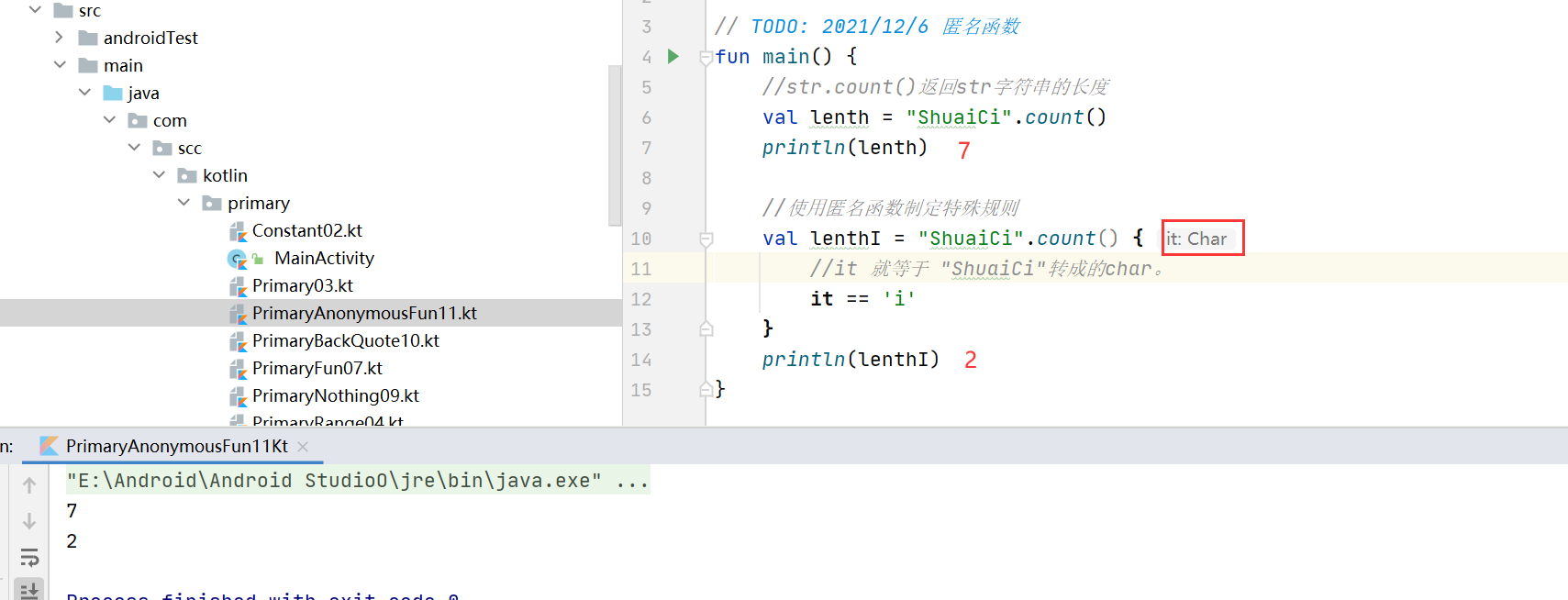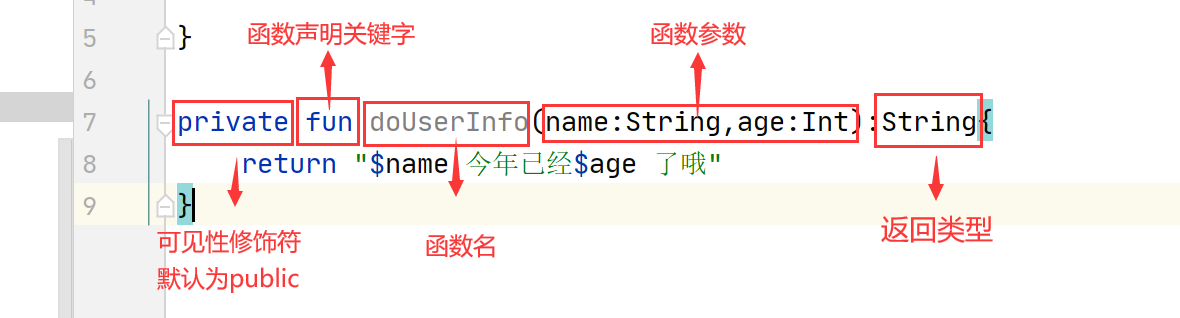+关注继续查看

# 《Kotlin极简教程》正式上架：

## 8.2 在Kotlin中使用函数式编程

### 8.2.1 Kotlin中的函数

#### 函数声明

Kotlin 中的函数使用 fun 关键字声明

fun double(x: Int): Int {
return 2*x
}


#### 函数用法

fun test() {
val doubleTwo = double(2)
println("double(2) = $doubleTwo") }  输出：double(2) = 4 调用成员函数使用点表示法 object FPBasics { fun double(x: Int): Int { return 2 * x } fun test() { val doubleTwo = double(2) println("double(2) =$doubleTwo")
}
}

fun main(args: Array<String>) {
FPBasics.test()
}


### 8.2.2 扩展函数

    fun String.swap(index1: Int, index2: Int): String {
val charArray = this.toCharArray()
val tmp = charArray[index1]
charArray[index1] = charArray[index2]
charArray[index2] = tmp

return charArrayToString(charArray)
}

fun charArrayToString(charArray: CharArray): String {
var result = ""
charArray.forEach { it -> result = result + it }
return result
}



        val str = "abcd"
val swapStr = str.swap(0, str.lastIndex)
println("str.swap(0, str.lastIndex) = $swapStr")  输出： str.swap(0, str.lastIndex) = dbca ### 8.2.3 中缀函数 在以下场景中，函数还可以用中缀表示法调用： • 成员函数或扩展函数 • 只有一个参数 • infix 关键字标注 例如，给 Int 定义扩展 infix fun Int.shl(x: Int): Int { ... }  用中缀表示法调用扩展函数： 1 shl 2  等同于这样 1.shl(2)  ### 8.2.4 函数参数 函数参数使用 Pascal 表示法定义，即 name: type。参数用逗号隔开。每个参数必须显式指定其类型。  fun powerOf(number: Int, exponent: Int): Int { return Math.pow(number.toDouble(), exponent.toDouble()).toInt() }  测试代码：  val eight = powerOf(2, 3) println("powerOf(2,3) =$eight")



#### 默认参数

    fun add(x: Int = 0, y: Int = 0): Int {
return x + y
}


        val zero = add()
println("add() = $zero") println("add(1) =$one")
println("add(1, 1) = $two")  输出： add() = 0 add(1) = 1 add(1, 1) = 2 另外，覆盖带默认参数的函数时，总是使用与基类型方法相同的默认参数值。 当覆盖一个带有默认参数值的方法时，签名中不带默认参数值： open class DefaultParamBase { open fun add(x: Int = 0, y: Int = 0): Int { return x + y } } class DefaultParam : DefaultParamBase() { override fun add(x: Int, y: Int): Int { // 不能有默认值 return super.add(x, y) } }  #### 命名参数 可以在调用函数时使用命名的函数参数。当一个函数有大量的参数或默认参数时这会非常方便。 给定以下函数  fun reformat(str: String, normalizeCase: Boolean = true, upperCaseFirstLetter: Boolean = true, divideByCamelHumps: Boolean = false, wordSeparator: Char = ' ') { }  我们可以使用默认参数来调用它 reformat(str)  然而，当使用非默认参数调用它时，该调用看起来就像 reformat(str, true, true, false, '_')  使用命名参数我们可以使代码更具有可读性 reformat(str, normalizeCase = true, upperCaseFirstLetter = true, divideByCamelHumps = false, wordSeparator = '_' )  并且如果我们不需要所有的参数 reformat(str, wordSeparator = '_')  #### 可变数量的参数（Varargs） 函数的参数（通常是最后一个）可以用 vararg 修饰符标记： fun <T> asList(vararg ts: T): List<T> { val result = ArrayList<T>() for (t in ts) // ts is an Array result.add(t) return result }  允许将可变数量的参数传递给函数： val list = asList(1, 2, 3)  ### 8.2.5 函数返回类型 #### 函数返回类型需要显式声明 具有块代码体的函数必须始终显式指定返回类型，除非他们旨在返回 Unit Kotlin 不推断具有块代码体的函数的返回类型，因为这样的函数在代码体中可能有复杂的控制流，并且返回类型对于读者（有时对于编译器）也是不明显的。 #### 返回 Unit 的函数 如果一个函数不返回任何有用的值，它的返回类型是 UnitUnit 是一种只有一个Unit 值的类型。这个值不需要显式返回:  fun printHello(name: String?): Unit { if (name != null) println("Hello${name}")
else
println("Hi there!")
// return Unit 或者 return 是可选的
}


Unit 返回类型声明也是可选的。上面的代码等同于

fun printHello(name: String?) {
.....
}


### 8.2.6 单表达式函数

fun double(x: Int): Int = x * 2


fun double(x: Int) = x * 2


### 8.2.7 函数作用域

#### 局部函数（嵌套函数）

Kotlin 支持局部函数，即一个函数在另一个函数内部

     fun sum(x: Int, y: Int, z: Int): Int {
val delta = 0;
fun add(a: Int, b: Int): Int {
return a + b + delta
}
}


println("sum(1,2,3) = ${sum(0, 1, 2, 3)}")  输出： sum(1,2,3) = 6 #### 成员函数 成员函数是在类或对象内部定义的函数 class Sample() { fun foo() { print("Foo") } }  成员函数以点表示法调用 Sample().foo() // 创建类 Sample 实例并调用 foo  ### 8.2.8 泛型函数 函数可以有泛型参数，通过在函数名前使用尖括号指定。 例如Iterable的map函数： public inline fun <T, R> Iterable<T>.map(transform: (T) -> R): List<R> { return mapTo(ArrayList<R>(collectionSizeOrDefault(10)), transform) }  ### 8.2.9 高阶函数 高阶函数是将函数用作参数或返回值的函数。例如，Iterable的filter函数： public inline fun <T> Iterable<T>.filter(predicate: (T) -> Boolean): List<T> { return filterTo(ArrayList<T>(), predicate) }  它的输入参数predicate: (T) -> Boolean就是一个函数。其中，函数类型声明的语法是： (X)->Y  表示这个函数是从类型X到类型Y的映射。即这个函数输入X类型，输出Y类型。 这个函数我们这样调用：  fun isOdd(x: Int): Boolean { return x % 2 == 1 } val list = listOf(1, 2, 3, 4, 5) list.filter(::isOdd)  其中，::用来引用一个函数。 ### 8.2.10 匿名函数 我们也可以使用匿名函数来实现这个predicate函数：  list.filter((fun(x: Int): Boolean { return x % 2 == 1 }))  ### 8.2.11 Lambda 表达式 我们也可以直接使用更简单的Lambda表达式来实现一个predicate函数：  list.filter { it % 2 == 1 }  • lambda 表达式总是被大括号 {} 括着 • 其参数（如果有的话）在 -> 之前声明（参数类型可以省略） • 函数体（如果存在的话）在 -> 后面 上面的写法跟：  list.filter({ it % 2 == 1 })  等价，如果 lambda 是该调用的唯一参数，则调用中的圆括号可以省略。 使用Lambda表达式定义一个函数字面值： >>> val sum = { x: Int, y: Int -> x + y } >>> sum(1,1) 2  我们在使用嵌套的Lambda表达式来定义一个柯里化的sum函数： >>> val sum = {x:Int -> {y:Int -> x+y }} >>> sum (kotlin.Int) -> (kotlin.Int) -> kotlin.Int >>> sum(1)(1) 2  ### 8.2.11 it：单个参数的隐式名称 Kotlin中另一个有用的约定是，如果函数字面值只有一个参数， 那么它的声明可以省略（连同 ->），其名称是 it 代码示例： >>> val list = listOf(1,2,3,4,5) >>> list.map { it * 2 } [2, 4, 6, 8, 10]  ### 8.2.12 闭包（Closure） Lambda 表达式或者匿名函数，以及局部函数和对象表达式（object declarations）可以访问其 闭包 ，即在外部作用域中声明的变量。 与 Java 不同的是可以修改闭包中捕获的变量：  fun sumGTZero(c: Iterable<Int>): Int { var sum = 0 c.filter { it > 0 }.forEach { sum += it } return sum } val list = listOf(1, 2, 3, 4, 5) sumGTZero(list) // 输出 15  我们再使用闭包来写一个使用Java中的Thread接口的例子：  fun closureDemo() { Thread({ for (i in 1..10) { println("I =$i")
}

}).start()

for (j in 10..20) {
println("J = \$j")
}
}).start()
}


I = 1
J = 10
I = 2
I = 3
...
J = 20


### 8.2.13 带接收者的函数字面值

Kotlin 提供了使用指定的 接收者对象 调用函数字面值的功能。

>>> val sum = fun Int.(other: Int): Int = this + other
>>> 1.sum(1)
2


class HTML {
fun body() {
println("HTML BODY")
}
}

fun html(init: HTML.() -> Unit): HTML { // HTML.()中的HTML是接受者类型
val html = HTML()  // 创建接收者对象
html.init()        // 将该接收者对象传给该 lambda
return html
}


    html {
body()
}



### 8.2.14 具体化的类型参数

fun <T> TreeNode.findParentOfType(clazz: Class<T>): T? {
var p = parent
while (p != null && !clazz.isInstance(p)) {
p = p.parent
}
@Suppress("UNCHECKED_CAST")
return p as T?
}


treeNode.findParentOfType(MyTreeNode::class.java)


treeNode.findParentOfType<MyTreeNode>()


inline fun <reified T> TreeNode.findParentOfType(): T? {
var p = parent
while (p != null && p !is T) {
p = p.parent
}
return p as T?
}


inline fun <reified T> membersOf() = T::class.members

fun main(s: Array<String>) {
println(membersOf<StringBuilder>().joinToString("\n"))
}


### 8.2.10 尾递归tailrec

Kotlin 支持一种称为尾递归的函数式编程风格。 这允许一些通常用循环写的算法改用递归函数来写，而无堆栈溢出的风险。 当一个函数用 tailrec 修饰符标记并满足所需的形式时，编译器会优化该递归，生成一个快速而高效的基于循环的版本。

tailrec fun findFixPoint(x: Double = 1.0): Double
= if (x == Math.cos(x)) x else findFixPoint(Math.cos(x)) // 函数必须将其自身调用作为它执行的最后一个操作



private fun findFixPoint(): Double {
var x = 1.0
while (true) {
val y = Math.cos(x)
if (x == y) return y
x = y
}
}


Kotlin 还为集合类引入了许多扩展函数。例如，使用 map() 和 filter() 函数可以流畅地操纵数据，具体的函数的使用以及示例我们已经在 集合类 章节中介绍。

## 本章小结|
2月前
|

AI问答：OOP （面向对象编程）、FP （函数式编程）、 FRP （函数响应式编程）、PP（面向过程编程）
AI问答：OOP （面向对象编程）、FP （函数式编程）、 FRP （函数响应式编程）、PP（面向过程编程）
50 0
|
4月前
|

61 0
|
7月前
|
Java 编译器
「 Java基础-Lambda 」试试Lambda表达式？通俗易懂得嘞!

48 1|
Java Kotlin
【Kotlin 初学者】函数(方法)（下）

108 0|
Java Android开发 Kotlin
【Kotlin 初学者】函数(方法)（上）

118 0|

《Kotin 极简教程》第8章 函数式编程（FP）(1)

1334 0
|
API

1216 0
|
Java
《Kotlin 程序设计》第六章 Kotlin 函数式编程（FP）

1184 0